Updating search results...

# 17 Results

View
Selected filters:
• MCCRS.Math.Content.6.G.A.2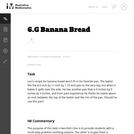Unrestricted Use
CC BY
Rating
0.0 stars

The purpose of this task is to provide students with a multi-step problem involving volume and to give them a chance to discuss the difference between exact calculations and their meaning in a context.

Subject:
Geometry
Mathematics
Material Type:
Activity/Lab
Provider:
Illustrative Mathematics
Provider Set:
Illustrative Mathematics
Author:
Illustrative Mathematics
05/01/2012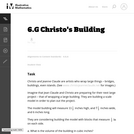Unrestricted Use
CC BY
Rating
0.0 stars

This task is primarily about volume and surface area, although it also gives students an early look at converting between measurements in scale models and the real objects they correspond to.

Subject:
Geometry
Mathematics
Material Type:
Activity/Lab
Provider:
Illustrative Mathematics
Provider Set:
Illustrative Mathematics
Author:
Illustrative Mathematics
05/01/2012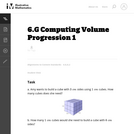Unrestricted Use
CC BY
Rating
0.0 stars

The purpose of this series of tasks is to build in a natural way from accessible, concrete problems involving volume to a more abstract understanding of volume. The purpose of this first task is to see the relationship between the side-lengths of a cube and its volume.

Subject:
Geometry
Mathematics
Material Type:
Activity/Lab
Provider:
Illustrative Mathematics
Provider Set:
Illustrative Mathematics
Author:
Illustrative Mathematics
05/01/2012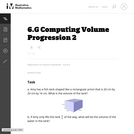Unrestricted Use
CC BY
Rating
0.0 stars

The purpose of this series of tasks is to build in a natural way from accessible, concrete problems involving volume to a more abstract understanding of volume. In this iteration, we do away with the lines that delineate individual unit cubes (which makes it more abstract) and generalize from cubes to rectangular prisms.

Subject:
Geometry
Mathematics
Material Type:
Activity/Lab
Provider:
Illustrative Mathematics
Provider Set:
Illustrative Mathematics
Author:
Illustrative Mathematics
05/01/2012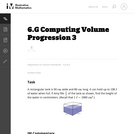Unrestricted Use
CC BY
Rating
0.0 stars

The purpose of this series of tasks is to build in a natural way from accessible, concrete problems involving volume to a more abstract understanding of volume. Here, we are given the volume and are asked to find the height.

Subject:
Geometry
Mathematics
Material Type:
Activity/Lab
Provider:
Illustrative Mathematics
Provider Set:
Illustrative Mathematics
Author:
Illustrative Mathematics
05/01/2012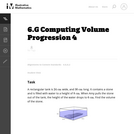Unrestricted Use
CC BY
Rating
0.0 stars

The purpose of this series of tasks is to build in a natural way from accessible, concrete problems involving volume to a more abstract understanding of volume. This problem is based on ArchimedesŐ Principle that the volume of an immersed object is equivalent to the volume of the displaced water.

Subject:
Geometry
Mathematics
Material Type:
Activity/Lab
Provider:
Illustrative Mathematics
Provider Set:
Illustrative Mathematics
Author:
Illustrative Mathematics
05/01/2012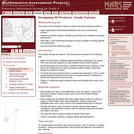Only Sharing Permitted
CC BY-NC-ND
Rating
0.0 stars

This lesson unit is intended to help teachers assess how well students are able to: Select appropriate mathematical methods to use for an unstructured problem; interpret a problem situation, identifying constraints and variables, and specify assumptions; work with 2- and 3-dimensional shapes to solve a problem involving capacity and surface area; and communicate their reasoning clearly.

Subject:
Geometry
Mathematics
Material Type:
Assessment
Lesson Plan
Provider:
Shell Center for Mathematical Education
Provider Set:
Mathematics Assessment Project (MAP)
04/26/2013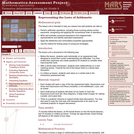Only Sharing Permitted
CC BY-NC-ND
Rating
0.0 stars

This lesson unit is intended to help you assess how well students are able to: Perform arithmetic operations, including those involving whole-number exponents, recognizing and applying the conventional order of operations; Write and evaluate numerical expressions from diagrammatic representations and be able to identify equivalent expressions; apply the distributive and commutative properties appropriately; and use the method for finding areas of compound rectangles.

Subject:
Geometry
Mathematics
Material Type:
Assessment
Lesson Plan
Provider:
Shell Center for Mathematical Education
Provider Set:
Mathematics Assessment Project (MAP)
04/26/2013Conditional Remix & Share Permitted
CC BY-NC
Rating
0.0 stars
Rating
0.0 stars
Subject:
Mathematics
Material Type:
Full Course
Provider:
Pearson
10/06/2016Conditional Remix & Share Permitted
CC BY-NC
Rating
0.0 stars

Surface Area and Volume

Type of Unit: Conceptual

Prior Knowledge

Students should be able to:

Identify rectangles, parallelograms, trapezoids, and triangles and their bases and heights.
Identify cubes, rectangular prisms, and pyramids and their faces, edges, and vertices.
Understand that area of a 2-D figure is a measure of the figure's surface and that it is measured in square units.
Understand volume of a 3-D figure is a measure of the space the figure occupies and is measured in cubic units.

Lesson Flow

The unit begins with an exploratory lesson about the volumes of containers. Then in Lessons 2–5, students investigate areas of 2-D figures. To find the area of a parallelogram, students consider how it can be rearranged to form a rectangle. To find the area of a trapezoid, students think about how two copies of the trapezoid can be put together to form a parallelogram. To find the area of a triangle, students consider how two copies of the triangle can be put together to form a parallelogram. By sketching and analyzing several parallelograms, trapezoids, and triangles, students develop area formulas for these figures. Students then find areas of composite figures by decomposing them into familiar figures. In the last lesson on area, students estimate the area of an irregular figure by overlaying it with a grid. In Lesson 6, the focus shifts to 3-D figures. Students build rectangular prisms from unit cubes and develop a formula for finding the volume of any rectangular prism. In Lesson 7, students analyze and create nets for prisms. In Lesson 8, students compare a cube to a square pyramid with the same base and height as the cube. They consider the number of faces, edges, and vertices, as well as the surface area and volume. In Lesson 9, students use their knowledge of volume, area, and linear measurements to solve a packing problem.

Subject:
Geometry
Mathematics
Material Type:
Unit of Study
Provider:
Pearson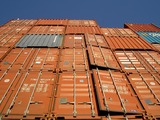Conditional Remix & Share Permitted
CC BY-NC
Rating
0.0 stars

Lesson OverviewStudents make two different rectangular prisms by folding two 812 in. by 11 in. sheets of paper in different ways. Then students use reasoning to compare the total areas of the faces of the two prisms (i.e., their surface areas). Students also predict how the amounts of space inside the prisms (i.e., their volumes) compare. They will check their predictions in Lesson 6.Key ConceptsStudents compare the total area of the faces (i.e., surface area) of one rectangular prism to the total area of the faces of another prism. Students make predictions about which prism has the greater amount of space inside (i.e., the greater volume). Students do not compute actual surface areas or volumes. This exploration helps pave the way for a more formal study of volume in Lesson 6 and a more formal study of surface area in Lesson 7.Goals and Learning ObjectivesExplore how the surface areas and volumes of two different prisms made from the same-sized sheet of paper compare.

Subject:
Geometry
Material Type:
Lesson Plan
09/21/2015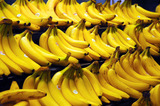Conditional Remix & Share Permitted
CC BY-NC
Rating
0.0 stars

Lesson OverviewStudents revise their packing plans based on teacher feedback and then take a quiz.Students will use their knowledge of volume, area, and linear measurements to solve problems. They will draw diagrams to help them solve a problem and track and review their choice of problem-solving strategies.Key ConceptsConcepts from previous lessons are integrated into this assessment task: finding the volume of rectangular prisms. Students apply their knowledge, review their work, and make revisions based on feedback from the teacher and their peers. This process creates a deeper understanding of the concepts.Goals and Learning ObjectivesApply your knowledge of the volume of rectangular prisms.Track and review your choice of strategy when problem-solving.

Subject:
Geometry
Material Type:
Lesson Plan
09/21/2015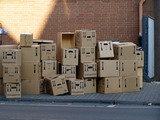Conditional Remix & Share Permitted
CC BY-NC
Rating
0.0 stars

Lesson OverviewStudents build prisms with fractional side lengths by using unit-fraction cubes (i.e., cubes with side lengths that are unit fractions, such as 13 unit or 14 unit). Students verify that the volume formula for rectangular prisms, V = lwh or V = bh, applies to prisms with side lengths that are not whole numbers.Key ConceptsIn fifth grade, students found volumes of prisms with whole-number dimensions by finding the number of unit cubes that fit inside the prisms. They found that the total number of unit cubes required is the number of unit cubes in one layer (which is the same as the area of the base) times the number of layers (which is the same as the height). This idea was generalized as V = lwh, where l, w, and h are the length, width, and height of the prism, or as V = Bh, where B is the area of the base of the prism and h is the height.Unit cubes in each layer = 3 &times; 4Number of layers = 5Total number of unit cubes = 3 &times; 4 &times; 5 = 60Volume = 60 cubic unitsIn this lesson, students extend this idea to prisms with fractional side lengths. They build prisms using unit-fraction cubes. The volume is the number of unit-fraction cubes in the prism times the volume of each unit-fraction cube. Students show that this result is the same as the volume found by using the formula.For example, you can build a 45-unit by 35-unit by 25-unit prism using 15-unit cubes. This requires 4 &times; 3 &thinsp;&times; 2, or 24, 15-unit cubes. Each 15-unit cube has a volume of 1125 cubic unit, so the total volume is 24125 cubic units. This is the same volume obtained by using the formula V = lwh:V=lwh=45×35×25=24125.15-unit cubes in each layer = 3 &times; 4Number of layers = 2Total number of 15-unit cubes = 3 &times; 4 &times; 2 = &thinsp;24Volume = 24 &times; 1125 = 24125 cubic units&nbsp;Goals and Learning ObjectivesVerify that the volume formula for rectangular prisms, V = lwh or V = Bh, applies to prisms with side lengths that are not whole numbers.

Subject:
Geometry
Material Type:
Lesson Plan
09/21/2015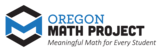Unrestricted Use
CC BY
Rating
0.0 stars

The intent of clarifying statements is to provide additional guidance for educators to communicate the intent of the standard to support the future development of curricular resources and assessments aligned to the 2021 math standards.&nbsp; Clarifying statements can be in the form of succinct sentences or paragraphs that attend to one of four types of clarifications: (1) Student Experiences; (2) Examples; (3) Boundaries; and (4) Connection to Math Practices.

Subject:
Mathematics
Material Type:
Teaching/Learning Strategy
Author:
Mark Freed
07/10/2023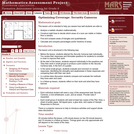Only Sharing Permitted
CC BY-NC-ND
Rating
0.0 stars

This lesson unit is intended to help sixth grade teachers assess how well students are able to: Analyze a realistic situation mathematically; construct sight lines to decide which areas of a room are visible or hidden from a camera; find and compare areas of triangles and quadrilaterals; and calculate and compare percentages and/or fractions of areas.

Subject:
Geometry
Mathematics
Ratios and Proportions
Material Type:
Assessment
Lesson Plan
Provider:
Shell Center for Mathematical Education
Provider Set:
Mathematics Assessment Project (MAP)
04/26/2013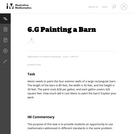Unrestricted Use
CC BY
Rating
0.0 stars

The purpose of this task is to provide students an opportunity to use mathematics addressed in different standards in the same problem.

Subject:
Geometry
Mathematics
Material Type:
Activity/Lab
Provider:
Illustrative Mathematics
Provider Set:
Illustrative Mathematics
Author:
Illustrative Mathematics
05/01/2012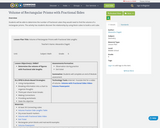Unrestricted Use
CC BY
Rating
0.0 stars

Students will be able to determine the number of fractional cubes they would need to find the volume of a rectangular prisms. The activity has students discover the relationship by using block cubes to build a unit cube.&nbsp;

Subject:
Geometry
Material Type:
Lesson Plan
Author:
Alexandra Clagett